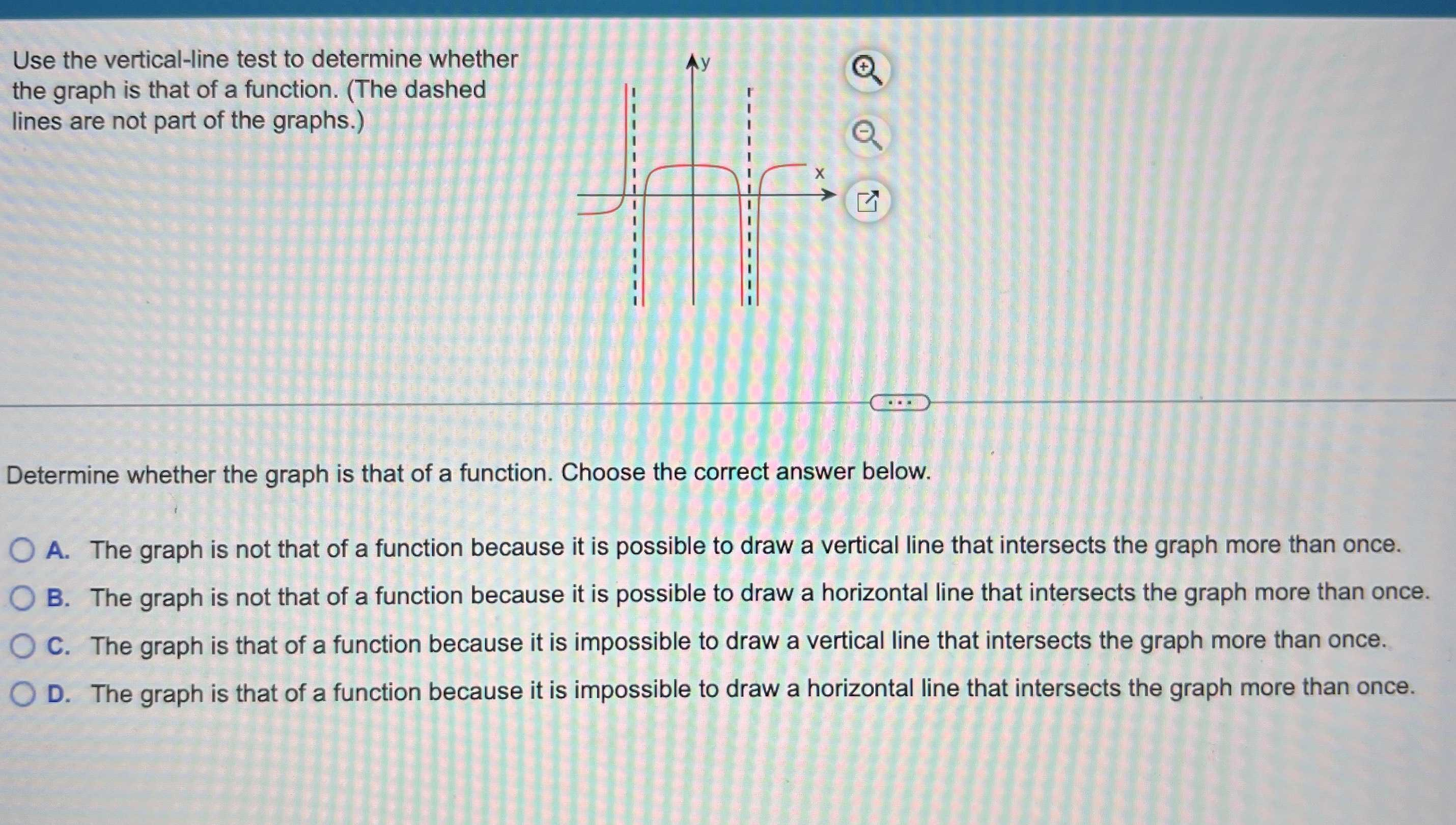### Still have math questions?

Algebra
QuestionUse the vertical line test to determine whether the graph is that of a function. (The dashed lines are not part of the graphs.)

Determine whether the graph is that of a function. Choose the correct answer below.

A. The graph is not that of a function because it is possible to draw a vertical line that intersects the graph more than once.

B. The graph is not that of a function because it is possible to draw a horizontal line that intersects the graph more than once.

C. The graph is that of a function because it is impossible to draw a vertical line that intersects the graph more than once.

D. The graph is that of a function because it is impossible to draw a horizontal line that intersects the graph more than once.